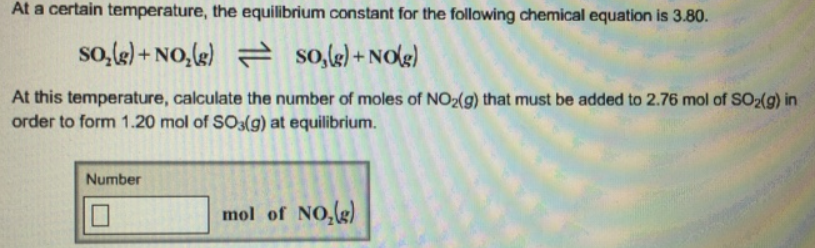# Problem: At a certain temperature, the equilibrium constant for the following chemical equation is 3.80. SO2 (g) + NO2 (g) ⇌ SO3 (g) + NO (g) At this temperature, calculate the number of moles of NO 2 (g) that must be added to 2.76 mol of SO2 (g) in order to form 1.20 mol of SO 3 (g) at equilibrium.

###### FREE Expert Solution
97% (270 ratings)###### Problem Details

At a certain temperature, the equilibrium constant for the following chemical equation is 3.80.

SO2 (g) + NO(g) ⇌ SO3 (g) + NO (g)

At this temperature, calculate the number of moles of NO (g) that must be added to 2.76 mol of SO2 (g) in order to form 1.20 mol of SO 3 (g) at equilibrium.